Courses

# Integer Answer Type Questions: Differentiation | JEE Advanced Notes | EduRev

## JEE : Integer Answer Type Questions: Differentiation | JEE Advanced Notes | EduRev

The document Integer Answer Type Questions: Differentiation | JEE Advanced Notes | EduRev is a part of the JEE Course Maths 35 Years JEE Mains & Advance Past year Papers Class 12.
All you need of JEE at this link: JEE

Assertion & Reason Type Question

Q. 1. Let f (x) = 2 + cos x for all real x.

STATEMENT - 1 : For each real t, there exists a point c in [t, t + π] such that f '(c) = 0 because
STATEMENT - 2 : f(t) = f(t + 2π) for each real t.

(a) Statement-1 is True, Statement-2 is True; Statement-2 is a correct explanation for Statement-1
(b) Statement-1 is True, Statement-2 is True; Statement-2 is NOT a correct explanation for Statement-1
(c) Statement-1 is True, Statement-2 is False
(d) Statement-1 is False, Statement-2 is True.

Ans. (b)

Solution. Given that f (x) = 2 + cos x which is continuous and differentiable every where.
Also f ' (x) = – sin x   ⇒  f ' (x) = 0  ⇒ x = nπ
⇒ There exists c ∈ [t, t + p] for t ∈ R
Such that f ' (c) = 0
∴ Statement-1 is true.
Also f (x) being periodic of period 2π, statement-2 is true, but statement-2 is not a correct explanation of statement-1.

Q. 2. Let f and g be real valued functions defined on interval (–1, 1) such that g" (x) is continuous, g (0) ≠ 0. g'(0) = 0, g ''(0) ≠ 0 , and f (x) = g (x) sin x

STATEMENT - 1 :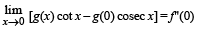and

STATEMENT - 2 : f '(0) = g(0)

(a) Statement - 1 is True, Statement - 2 is True; Statement
- 2 is a correct explanation for Statement - 1
(b) Statement - 1 is True, Statement - 2 is True; Statement
- 2 is NOT a correct explaination for Statement - 1
(c) Statement - 1 is True, Statement - 2 is False
(d) Statement - 1 is False, Statement - 2 is True

Ans. (a)

Solution. We have f (x) = g(x) sin x
⇒ f '(x) = g'(x) sin x + g(x) cos x
⇒ f '(0) = g'(0) × 0 + g(0) = g(0)    [∴ g'(0) = 0]
∴ Statement 2 is correct.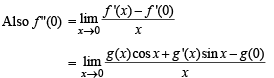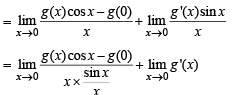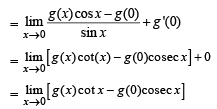∴ Statement 1 is also true and is a correct explanation for statement 2.

Integer Value Correct Type Question

Q. 1. If the function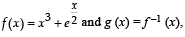then the value of g' (1) is

Ans. 2

Solution. Given that f (x) = x3+e x /2 and g (x) = f -1 (x) then we should have gof ( x) = x

⇒ g(f (x)) = x ⇒ g(x3 +ex/2) = x

Differentiating both sides with respect to x, we get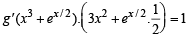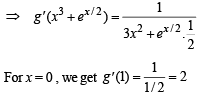Q. 2.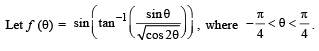Then the value of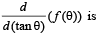Ans. 1

Solution.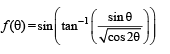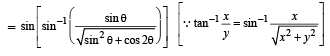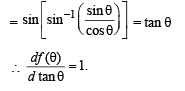Offer running on EduRev: Apply code STAYHOME200 to get INR 200 off on our premium plan EduRev Infinity!

63 docs|43 tests

,

,

,

,

,

,

,

,

,

,

,

,

,

,

,

,

,

,

,

,

,

;